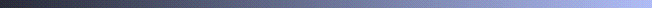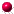## GSLIB Help Page: KT3DDescription:
• The program kt3d provides a fairly advanced 3-D kriging program for points or blocks by simple kriging (SK), ordinary kriging (OK), or kriging with a polynomial trend model (KT) with up to nine monomial terms. The program works in 2-D and is faster than kb2d if there are many data. One of the features that makes this program fairly fast is the super block search.Parameters:
• datafl: the input data in a simplified Geo-EAS formatted file.
• icolx, icoly, icolz, icolvr and icolsec: the columns for the x, y, and z coordinates, the variable to be estimated, and the external drift variable (or non-stationary mean).
• tmin and tmax: all values strictly less than tmin and greater than or equal to tmax are ignored.
• option: set to 0 for kriging a grid of points or blocks, to 1 for cross validation with the data in datafl and to 2 for jackknifing with data in following file.
• jackfl: file with locations to perform estimation (jackknife option).
• icolx, icoly, icolz, icolvr and icolsec: the columns for the x, y, and z coordinates, the variable, and the secondary variable in jackfl
• idbg: an integer debugging level between 0 and 3. The higher the debugging level the more output. The normal levels are 0 and 1 which summarize the results. Levels 2 and 3 provide all the kriging matrices and data used for the estimation of every point/block. It is recommended that a high debugging level not be used with a large grid.
• dbgfl: the debugging output is written to this file.
• outfl: the output grid is written to this file. The output contains the estimate and the kriging variance for every point/block on the grid, cycling fastest on x then y and finally z Unestimated points are flagged with a large negative number (-999.). The parameter UNEST, in the source code, can be changed if a different number is preferred.
• nx, xmn, xsiz: definition of the grid system (x axis).
• ny, ymn, ysiz: definition of the grid system (y axis).
• nz, zmn, zsiz: definition of the grid system (z axis).
• nxdis, nydis and nzdis: the number of discretization points for a block. If nxdis, nydis and nzdis are all set to 1 then point kriging is performed.
• ndmin and ndmax: the minimum and maximum number of data points to use for kriging a block.
• noct: the maximum number to retain from an octant (an octant search is not used if noct=0
• radius_hmax radius_hmin and radius_vert the search radii in the maximum horizontal direction, minimum horizontal direction, and vertical direction (see angles below).
• sang1, sang2 and sang3: the angle parameters that describe the orientation of the search ellipsoid. See the discussion on anisotropy specification associated with Figure II.4.
• ikrige and skmean: if ikrige is set to 0 then stationary simple kriging with (skmean) will be performed, if ikrige is set to 1 then ordinary kriging will be performed, if ikrige is set to 2 then non-stationary simple kriging with means taken from secfile will be performed, and if ikrige is set to 3 then kriging with an external drift will be performed. Note that power law variogram models (it=4) are not allowed with simple kriging.
• idrif(i),i=1...9: indicators for those drift terms to be included in the trend model. idrif(i) is set to 1 if the drift term number i should be included, and is set to zero if not. The nine drift terms correspond to the following:
• i = 1 linear drift in x
• i = 2 linear drift in y
• i = 3 linear drift in z
• i = 4 quadratic drift in x
• i = 5 quadratic drift in y
• i = 6 quadratic drift in z
• i = 7 cross quadratic drift in xy
• i = 8 cross quadratic drift in xz
• i = 9 cross quadratic drift in yz
• itrend: indicator of whether to estimate the trend (itrend =1) or the variable (itrend =0). The trend may be kriged with ordinary kriging (all idrif(i) values set to 0) or with any combination of trend kriging (some idrif(i) terms set to 1).
• secfl: a file for the gridded external drift variable. The external drift variable is needed at all grid locations to be estimated. The origin of the grid network, the number of nodes, and the spacing of the grid nodes should be exactly the same as the grid being kriged in kt3d This variable is used only if ikrige=2 or 3.
• iseccol: the column number in secfl for the gridded secondary variable. This variable is used if ikrige=2 or 3.
• nst and c0: the number of variogram structures and the nugget constant. The nugget constant does not count as a structure.
• For each of the nst nested structures one must define it, the type of structure; cc, the c parameter; ang1,ang2,ang3, the angles defining the geometric anisotropy; aa_hmax, the maximum horizontal range; aa_hmin, the minimum horizontal range; and aa_vert, the vertical range.Application notes:
• The program is set up so that a novice programmer can make changes to the form of the polynomial drift. The external drift concept has been incorporated, adding an additional unbiasedness constraint to the ordinary kriging system. When using an external drift, it is necessary to know the value of the drift variable at all data locations and all the locations that will be estimated (i.e., all grid nodes).
• The program also allows simple kriging with non-stationary means read from an input file. The non-stationary means must be known at all data locations and all locations to be estimated.